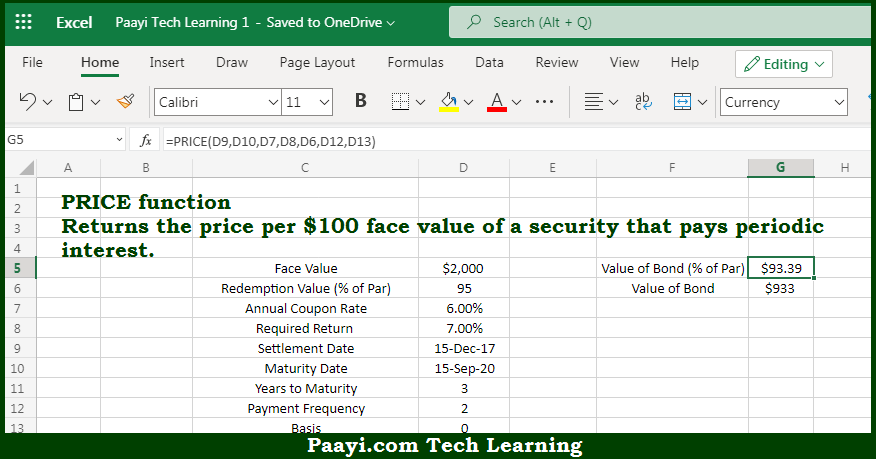# Learn How to Use Microsoft Excel PRICE Function

Written by | 0 Comments | 579 Views

In this article, you will learn how to use the Microsoft Excel PRICE function and its prime function in Microsoft Excel. You will also get to know the Microsoft Excel PRICE function return value and syntax with the help of some examples.

Microsoft Excel PRICE Function

The main purpose of the Microsoft Excel PRICE function is to get the price per \$100 face value of the periodic interest. That implies, with the help of the PRICE function you can able to return the price per \$100 face value of a security that pays periodic interest. So, with the help of the PRICE function, you can able to get the price per \$100 face value of the periodic interest.

Return Value of PRICE Function

The return value will be the bond price.

Syntax of PRICE Function

=PRICE(sd, md, rate, yld, redemption, frequency, [basis])

Where the arguments:

• sd: This is the settlement date of the security.
• md: This is the maturity date of the security.
• rate: This is the annual coupon rate.
• yld: This is the annual required rate of return.
• redemption: This is the redemption value per \$100 face value.
• frequency: This is the coupon payments per year, where annual = 1, semiannual = 2; quarterly = 4.
• basis: This is the day count basis, and defaults to 0 (optional).

How to Use Microsoft Excel PRICE Function?So we know that Microsoft Excel PRICE function you can able to get the price per \$100 face value of the periodic interest. That implies, with the help of the PRICE function you can able to return the price per \$100 face value of a security that pays periodic interest. So, with the help of the PRICE function, you can able to get the price per \$100 face value of the periodic interest.